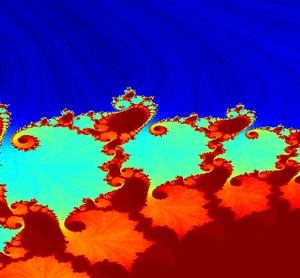The eleventh meeting of the Houston Recreational Computer Programming Group was held Sunday, November 10th, 2013.

Stephen Cameron and Jeremy Van Grinsven talked about how they used quaternions in the game Space Nerds in Space to represent the orientation of objects within the game as well as rotations and incrementally applied rotations. Quaternions are a mathematical construct represented by four floating point numbers, three of which encode an axis about which rotation occurs, while the fourth encodes the angle of rotation about that axis. If a vector, x, y, z represents the axis of rotation, and an angle, theta, represents the angle of rotation, the four components of the quaternion are computed as follows:

```    q = cos(theta / 2);
q = x * sin(theta / 2);
q = y * sin(theta / 2);
q = z * sin(theta / 2);
```

The details of how the encoding is done, or why it is done the way it is done are not necessary to understand in order to be able to use quaternions. Any accumulation of of any number of arbitrary rotations about a single point may be represented by a single quaternion.

What makes them useful in applications like computer games is that consecutive rotations may be accumulated by multiplying quaternions together. And a rotation may be transformed into the coordinate space of another arbitrarily rotated object easily.

For the latter, you need a quaternion representing the rotation to be transformed, and a quaternion representing the orientation of the coordinate system you which to transform the rotation into. Let's call them R and O, respectively. There is a "conjugate" operator defined for quaternions, let's call it C(x). To transform R into O's coordinate system, simply compute O * R * C(O) * O. In this way for example a spaceship's navigational controls affecting yaw, pitch, and roll in that ship's local coordinate system can be applied to the quaternion representing the ship's orientation. First combine the yaw, pitch and roll into a single quaternion via quaternion multiplication, and then this combined rotation is transformed into the ship's local coordinate system by computing O * R * C(O). Then that result is applied by multiplication with the ship's orientation quaternion. This all sounds horribly complicated, but with some fairly simple to write functions which can be found online to:

• Multiply a vector by a quaterion (rotates the vector)
• Multiply a quaternion by a quaternion
• Compute the conjugate of a quaternion
• Construct a quaternion given an axis vector and an angle
these functions are most of what you need to model the motions of say, a spaceship in a 3D space game.

Additionally, to do some interpolation to smooth animations and spread a rotation out over time between various orientations you may need functions to do spherical linear interpolation or normalized linear interpolation, and these are also easily looked up.